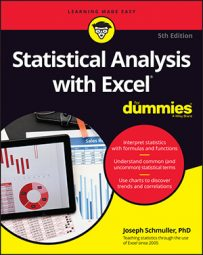##### Statistical Analysis with Excel For DummiesExcel provides two worksheet functions for calculating correlation — and, they do exactly the same thing in exactly the same way! Why Excel offers both `CORREL` and `PEARSON` is unclear, but there you have it. Those are the two main correlation functions.

The others are `RSQ`, `COVARIANCE.P`, and `COVARIANCE.S`. `RSQ` calculates the coefficient of determination (the square of the correlation coefficient). `COVARIANCE.P` determines covariance. It uses N-1. `COVARIANCE.S` uses N.

## CORREL and PEARSON

Here, you see an example of `CORREL` along with the Function Arguments dialog box for `CORREL`.

To use this function, follow these steps:

1. Type the data into cell arrays and select a cell for `CORREL`’s answer. Enter the SAT data into C3:C22 and the GPA data into D3:D22, and selected F15. SAT has been defined as the name of C3:C22 and GPA as the name of D3:D22.
2. From the Statistical Functions menu, select CORREL to open its Function Arguments dialog box.
3. In the Function Arguments dialog box, enter the appropriate values for the arguments. In the Array1 box, enter `SAT` — the name assigned to the cell range (C3:C22) that holds the scores for one of the variables. In the Array2 box, enter `GPA` — the name assigned to the cell range (D3:D22) that holds the scores for the other variable. With values entered for each argument, the answer, 0.81662505, appears in the dialog box.
4. Click OK to put the answer into the selected cell. Selecting `PEARSON` instead of `CORREL` gives you exactly the same answer, and you use it exactly the same way.

## RSQ

If you have to quickly calculate the coefficient of determination (r2), `RSQ` is the function for you. There really is no particular need for this function because it’s easy enough to use `CORREL` and then square the answer.

Here’s what the Excel Formula bar looks like after you fill in the RSQ Function Arguments dialog box for this example:

`=RSQ(GPA,SAT)`

In terms of the dialog box, the only difference between this one and `CORREL` (and `PEARSON`) is that the boxes you fill in are called Known_y's and Known_x's rather than Array1 and Array2.

## COVARIANCE.P and COVARIANCE.S

As far as calculating correlations go, there is really no need for these formulas.

You use these functions the same way you use `CORREL`. After you fill in the Function Arguments dialog box for `COVARIANCE.P` for this example, the formula in the Formula bar is

`=COVARIANCE.P(SAT,GPA)`

If you want to use this function to calculate r, you divide the answer by the product of `STDEV.P(SAT)` and `STDEV.P(GPA)`. It’s up to you to figure out how you’d use `COVARIANCE.S` to calculate r. It’s unclear why you’d bother with all of this when you can just use `CORREL`.